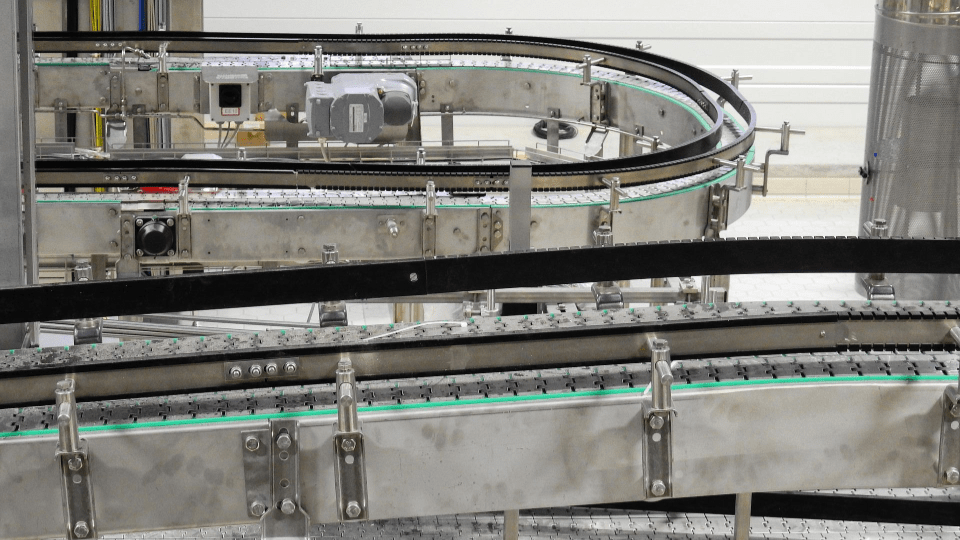# Conveyor routing simulation in AnyLogicHaving introduced AnyLogic with various smaller examples on this blog I here post a summary of a simulation model example published on The AnyLogic Modeler. I show how AnyLogic can be used for assessing conveyor layout variants. You can find a more comprehensive article on this conveyor routing simulation here: Conveyor simulation with material routings in AnyLogic (theanylogicmodeler.com).

## Conveyor routing simulation vs. analytical optimization

In other articles I already discussed my preferred simulation study procedure model. Moreover, I also introduced a workflow for conveyor system optimization. My workflow recommends that any conveyor system should first be formulated as a mathematical program. Iterative layout adjustments should, to begin with, be reflected in the mathematical program. Solving the mathematical program(s) will indicate maximum throughput capacities and optimal routing ratios and/or routing rules.

Next, a dynamic simulation model should be developed and deployed. Using the simulation model the effects of dynamic system behaviour and interdependencies can be assessed. A mathematical program cannot reflect complex system interdependencies resulting from dynamic equipment interactions.

Consequently, a dynamic simulation model should be used to verify analytically derived results. Below are some links to previous articles related to conveyor routing optimization and conveyor routing simulation.

Proceeding in this way implies that the simulation model considers the routing rules derived from the analytically solved mathematical program. I refer to this as conveyor routing simulation.

## Exemplary conveyor routing simulation in AnyLogic

An overview of an exemplary conveyor routing simulation can be seen in below screenshot. This screenshot is taken directy from AnyLogic. AnyLogic is a multi-purpose simulation software that e.g. allows for both agent-based simulation and discrete-event simulation modeling.

Essentially, the conveyor network receives inflows at five inlet conveyors and then routes material from there to either (a) one of two buffer areas, or (b) one of the processing stations at the end of the conveyor network. Depending on the part families being supplied from upstream production/supply processes vs. the ones requested by final processing (downstream) the buffer either builds up or reduces inventory of respective part family.

## Turntable utilization and balanced buffer fill rates

The simulation model balances fill rates of both buffer areas. A simulated fill rate curve can be seen below. The simulated fill rate curve also shows that both buffers “breathe”. That is, there are periods during which buffer inventory goes up (e.g. because one part family is supplied in excess of what is requested by final processing downstream) and periods during which inventory goes down (buffer supplies downstream final processing).

One other import aspect that had to be monitored was turntable utilization. Below chart shows the utilization of some important turntables in the original layout.

After adjusting the position of some of the inlet conveyors turntable utilization was much more balanced. This can be seen in the following chart.

Layout adjustments resulted in both critical turntables being utilized to the fullest. The result of this is a 13% increase in simulated conveyor network throughput.

## Concluding remarks on conveyor routing simulation

AnyLogic simulation modeling complements conveyor system optimization by facilitating dynamic system simulation. A dynamic AnyLogic simulation model considers dynamic system interactions. Analytical models, used ahead of simulation modeling for system drafting and routing optimization, cannot consider complex equipment interdependencies in the layout. Nevertheless they are appropriate for optimizing routing ratios ahead of simulation-based concept verification.

If you are interested in simulation modeling you might like some of the following articles: